# VCS Templates ¶

This turorial describes how to use CDAT's VCS's templates.

Download the Jupyter Notebook

The CDAT software was developed by LLNL. This tutorial was written by Charles Doutriaux (18 Jan. 2017). This work was performed under the auspices of the U.S. Department of Energy by Lawrence Livermore National Laboratory under Contract DE-AC52-07NA27344.

In the VCS model, the data display is defined by a trio of named object sets, designated the “primary objects” (or “primary elements”). These include:

• Data Ingestion: The data, which drives the visualization, is ingested into the system via cdms2 or other numeric modules such as numpy.
• Graphics Method: The graphics method, which specifies the display technique.
• Template: The picture template, which determines the appearance of each segment of the display. Tables for manipulating these primary objects are stored in VCS for later recall and possible use.

This tutorial explains how to control the template and its relation to VCS's other primary objects.

## Template elements¶

### Generalities¶

Back to top

The template object allows the user to control where various elements of the plots are being drawn.

The following picture shows the various elements of a VCS image and the associated VCS template's atrribute:To create a text object simply use the createtemplate function.

In :
from __future__ import print_function  # For Python 2 compatibility
import vcs
x = vcs.init(bg=True)
template = vcs.createtemplate()
# the name is some bad random name:
print("template name:", template.name)
# If you want you can name your template object for easier retrieval later
# but keep in mind the name must be unique
try:
template = vcs.createtemplate("vcs_is_easy")
except Exception:
print("Ooops already have a template named like this")

print("Nice named template:", template.name)
# fails on second try
try:
template = vcs.createtemplate("vcs_is_easy")
print("It worked")
except Exception:
print("Second try: Ooops already have a template named like this")


template name: __template_341325303621890
Nice named template: vcs_is_easy
Second try: Ooops already have a template named like this


You can also list all of your existing templates and use one of them as a starting point.

In :
mytemplates = vcs.listelements("template")
print("Existing templates:", mytemplates)  # notice the randomly named ones
template = vcs.createtemplate(source="vcs_is_easy")

Existing templates: ['ASD', 'ASD_dud', 'BL_of6_1legend', 'BLof6', 'BR_of6_1legend', 'BRof6', 'LLof4', 'LLof4_dud', 'LRof4', 'LRof4_dud', 'ML_of6', 'ML_of6_1legend', 'MR_of6', 'MR_of6_1legend', 'UL_of6_1legend', 'ULof4', 'ULof4_dud', 'ULof6', 'UR_of6', 'UR_of6_1legend', 'URof4', 'URof4_dud', '__template_341325303621890', 'bold_mid_of3', 'bold_top_of3', 'boldbot_of3_l', 'boldmid_of3_l', 'boldtop_of3_l', 'bot_of2', 'default', 'deftaylor', 'hovmuller', 'mollweide2', 'no_legend', 'polar', 'por_botof3', 'por_botof3_dud', 'por_midof3', 'por_midof3_dud', 'por_topof3', 'por_topof3_dud', 'quick', 'top_of2', 'vcs_is_easy']


Now in order to use this template either pass the template object to the vcs plot function.

In :
# Get some data to plot
vcs.download_sample_data_files()
import cdms2
import os
with cdms2.open(os.path.join(vcs.sample_data,"ta_ncep_87-6-88-4.nc")) as f:
data = f("ta")
# we need
x.plot(data, template)

MD5: /home/nadeau1/.cache/Python-Eggs/vcs-v8.1_9_g266121be-py3.7.egg-tmp/share/vcs/sample_files.txt
Downloading: 'th_yr.nc' from 'https://cdat.llnl.gov/cdat/sample_data/' in: /software/anaconda53/envs/cdms2/share/cdat/sample_data/th_yr.nc
Downloading: 'th_yr.nc' from 'https://cdat.llnl.gov/cdat/sample_data/' in: /software/anaconda53/envs/cdms2/share/cdat/sample_data/th_yr.nc
Downloading: 'th_yr.nc' from 'https://cdat.llnl.gov/cdat/sample_data/' in: /software/anaconda53/envs/cdms2/share/cdat/sample_data/th_yr.nc

Out: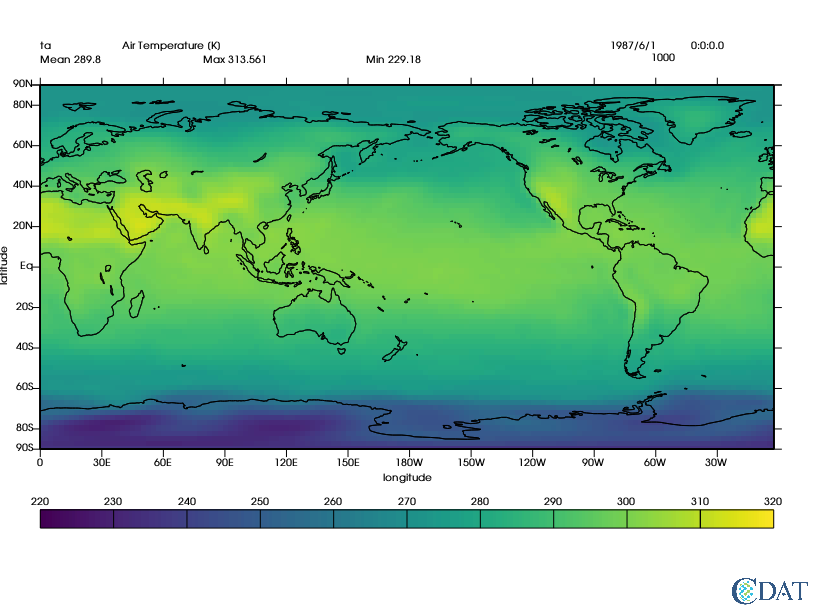Now let's learn how to customize template objects.

To see the list of available attributes on a template (and their own attributes/values) use the list() function.

In :
template.list()

---------- Template (P) member (attribute) listings ----------
method = P
name = __template_77340719414112
orientation = 0
member =  file
priority = 1
x = 0.0500000007451
y = 0.0130000002682
texttable = default
textorientation = default
member =  function
priority = 1
x = 0.0500000007451
y = 0.0130000002682
texttable = default
textorientation = default
member =  logicalmask
priority = 1
x = 0.0500000007451
y = 0.0329999998212
texttable = default
textorientation = default
member =  transformation
priority = 1
x = 0.0500000007451
y = 0.0529999993742
texttable = default
textorientation = default
member =  source
priority = 1
x = 0.0500000007451
y = 0.941999971867
texttable = default
textorientation = default
member =  dataname
priority = 1
x = 0.0500000007451
y = 0.922999978065
texttable = default
textorientation = default
member =  title
priority = 1
x = 0.15000000596
y = 0.922999978065
texttable = default
textorientation = default
member =  units
priority = 1
x = 0.670000016689
y = 0.922999978065
texttable = default
textorientation = default
member =  crdate
priority = 1
x = 0.75
y = 0.922999978065
texttable = default
textorientation = default
member =  crtime
priority = 1
x = 0.850000023842
y = 0.922999978065
texttable = default
textorientation = default
member =  comment1
priority = 1
x = 0.10000000149
y = 0.954999983311
texttable = default
textorientation = default
member =  comment2
priority = 1
x = 0.10000000149
y = 0.975000023842
texttable = default
textorientation = default
member =  comment3
priority = 1
x = 0.10000000149
y = 0.995000004768
texttable = default
textorientation = default
member =  comment4
priority = 1
x = 0.10000000149
y = 0.999000012875
texttable = default
textorientation = default
member =  xname
priority = 1
x = 0.5
y = 0.21
texttable = default
textorientation = defcenter
member =  yname
priority = 1
x = 0.006
y = 0.56
texttable = default
textorientation = defup
member =  zname
priority = 0
x = 0.0
y = 0.995000004768
texttable = default
textorientation = default
member =  tname
priority = 0
x = 0.0
y = 0.995000004768
texttable = default
textorientation = default
member =  xunits
priority = 0
x = 0.600000023842
y = 0.21
texttable = default
textorientation = default
member =  yunits
priority = 0
x = 0.006
y = 0.658999979496
texttable = default
textorientation = defup
member =  zunits
priority = 0
x = 0.0
y = 0.995000004768
texttable = default
textorientation = default
member =  tunits
priority = 0
x = 0.0
y = 0.995000004768
texttable = default
textorientation = default
member =  xvalue
priority = 0
x = 0.800000011921
y = 0.941999971867
format = default
texttable = default
textorientation = default
member =  yvalue
priority = 0
x = 0.800000011921
y = 0.922999978065
format = default
texttable = default
textorientation = default
member =  zvalue
priority = 1
x = 0.800000011921
y = 0.902999997139
format = default
texttable = default
textorientation = default
member =  tvalue
priority = 0
x = 0.800000011921
y = 0.883000016212
format = default
texttable = default
textorientation = default
member =  mean
priority = 1
x = 0.0500000007451
y = 0.899999976158
format = default
texttable = default
textorientation = default
member =  min
priority = 1
x = 0.449999988079
y = 0.899999976158
format = default
texttable = default
textorientation = default
member =  max
priority = 1
x = 0.25
y = 0.899999976158
format = default
texttable = default
textorientation = default
member =  xtic1
priority = 1
y1 = 0.259999990463
y2 = 0.24699999392
line = default
member =  xtic2
priority = 1
y1 = 0.860000014305
y2 = 0.871999979019
line = default
member =  xmintic1
priority = 0
y1 = 0.259999990463
y2 = 0.248999999285
line = default
member =  xmintic2
priority = 0
y1 = 0.860000014305
y2 = 0.868000014305
line = default
member =  ytic1
priority = 1
x1 = 0.0500000007451
x2 = 0.0399999991059
line = default
member =  ytic2
priority = 1
x1 = 0.949999988079
x2 = 0.959999978542
line = default
member =  ymintic1
priority = 0
x1 = 0.0500000007451
x2 = 0.0450000017881
line = default
member =  ymintic2
priority = 0
x1 = 0.949999988079
x2 = 0.954999983311
line = default
member =  xlabel1
priority = 1
y = 0.234999999404
texttable = default
textorientation = defcenter
member =  xlabel2
priority = 0
y = 0.880000004768
texttable = default
textorientation = defcenter
member =  ylabel1
priority = 1
x = 0.0399999991059
texttable = default
textorientation = defright
member =  ylabel2
priority = 0
x = 0.959999978542
texttable = default
textorientation = default
member =  box1
priority = 1
x1 = 0.0500000007451
y1 = 0.259999990463
x2 = 0.949999988079
y2 = 0.860000014305
line = default
member =  box2
priority = 0
x1 = 0.0
y1 = 0.300000011921
x2 = 0.920000016689
y2 = 0.879999995232
line = default
member =  box3
priority = 0
x1 = 0.0
y1 = 0.319999992847
x2 = 0.910000026226
y2 = 0.860000014305
line = default
member =  box4
priority = 0
x1 = 0.0
y1 = 0.0
x2 = 0.0
y2 = 0.0
line = default
member =  line1
priority = 0
x1 = 0.0500000007451
y1 = 0.560000002384
x2 = 0.949999988079
y2 = 0.560000002384
line = default
member =  line2
priority = 0
x1 = 0.5
y1 = 0.259999990463
x2 = 0.5
y2 = 0.860000014305
line = default
member =  line3
priority = 0
x1 = 0.0
y1 = 0.52999997139
x2 = 0.899999976158
y2 = 0.52999997139
line = default
member =  line4
priority = 0
x1 = 0.0
y1 = 0.990000009537
x2 = 0.899999976158
y2 = 0.990000009537
line = default
member =  legend
priority = 1
x1 = 0.0500000007451
y1 = 0.129999995232
x2 = 0.949999988079
y2 = 0.159999996424
line = default
texttable = default
textorientation = defcenter
offset = 0.01
member =  data
priority = 1
x1 = 0.0500000007451
y1 = 0.259999990463
x2 = 0.949999988079
y2 = 0.860000014305


### Data attribute¶

Back to top

One of the most important attributes is the data attribute. It controls where the data will be drawn.

Note that changing the data attribute alone is not sufficient; other attributes will need to be moved as well. See the latter part of this tutorial for utility functions to help you manipulate everything at once.

Let's try to move the data to the upper left part of the canvas.

All units are in percentage (%) of the canvas; the 0 representing the lower/left corner of the canvas and 1 the upper/right corner).

The data attribute has 5 sub-attributes:

• priority: the priority represents the layer on which the attribute will be drawn. A higher number means the attribute will be drawn on top of attributes with lower numbers.
• x1: The lower left corner horizontal location (in %).
• x2: The upper right corner horizontal location (in %).
• y1: The lower left corner vertical location (in %).
• y2: The upper right corner vertical location (in %).
In :
template.data.x1 = .1 # 10% from left
template.data.x2 = .45  # 45% from left
template.data.y1 = .45  # 45% from bottom
template.data.y2 = .85  # 15% from top (or 85% from bottom)

x.clear()
x.plot(data,template)

Out: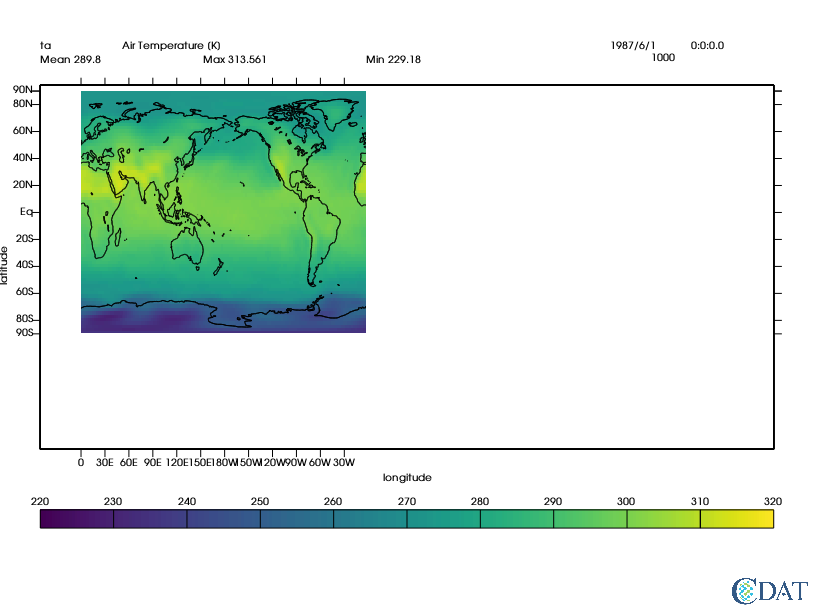As mentioned, other attributes need to be moved as well. First let's move the box around the data area. It is controlled via box1 which has the following attributes:

In :
template.box1.list()

member =  box1
priority = 1
x1 = 0.0500000007451
y1 = 0.259999990463
x2 = 0.949999988079
y2 = 0.860000014305
line = default


The new attribute here is the line attribute. You can use any line object from vcs (or create one).

In :
print(vcs.listelements("line"))
template.box1.line = "red"
# let's copy
template.box1.x1 = template.data.x1
template.box1.x2 = template.data.x2
template.box1.y1 = template.data.y1
template.box1.y2 = template.data.y2

x.clear()
x.plot(data,template)

['black', 'blue', 'brown', 'continents', 'cyan', 'default', 'deftaylordot', 'green', 'ltblue', 'navy', 'pink', 'purple', 'red', 'solid', 'std', 'thick', 'white']

Out: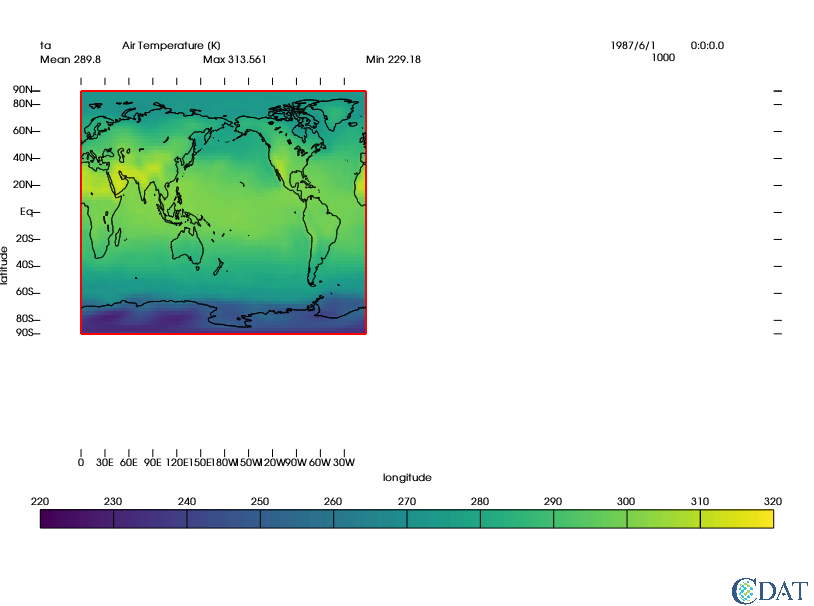### Tick Marks¶

Back to top

Now let's move the tick marks. There are two types of tick marks: "major" and "min". The main difference is that "major" tick marks have text associated with them.

There are tick marks for the x axis and for the y axis. Each axis has two sets of tick marks. By default 1 is for the left/bottom tick marks while 2 is for the right/top ones.

With this in mind, the tickmark attributes are:

xtic1, xtic2, xmintic1, xmintic2, ytic1, ytic2, ymintic1, ymintic2,

#### Major Ticks¶

Back to top

For example, for xtic1 the attributes are:

In :
template.xtic1.list()

member =  xtic1
priority = 1
y1 = 0.259999990463
y2 = 0.24699999392
line = default

• priority: the priority represents the layer on which the attribute will be drawn. A higher number means the attribute will be drawn on top of attributes with lower numbers.
• y1: start of tick line in the vertical direction (in % of canvas).
• y2: end of tick line in the vertical direction (in % of canvas).
• line: a vcs line object (or name) to use for the line.

Similarly for the y ticks:

In :
template.ytic1.list()

member =  ytic1
priority = 1
x1 = 0.0500000007451
x2 = 0.0399999991059
line = default

• priority: the priority represents the layer on which the attribute will be drawn. A higher number means the attribute will be drawn on top of attributes with lower numbers.
• x1: start of tick line in the horizontal direction (in % of canvas).
• x2: end of tick line in the horizontal direction (in % of canvas).
• line: a vcs line object (or name) to use for the line.

Note that a template only controls the extent of the tick marks, not their location/values. This is controlled via the graphic method's [x/y]ticlabels[1/2] and [x/y]mtics[1/2] attributes.

Let's set the tick marks properly.

##### First set of major ticks (x/y)¶
In :
# x major tick marks for first set of tick marks (1) drawn all the way across with grey lines
template.xtic1.y1 = template.data.y1
template.xtic1.y2 = template.data.y2
grey = vcs.createline()
grey.color = ['grey',]
grey.width = 
template.xtic1.line = grey  # uses line object

# y major for first set of tick marks (1) drawn all the way across with darker grey lines
template.ytic1.x1 = template.data.x1
template.ytic1.x2 = template.data.x2
grey2 = vcs.createline()
grey2.color = [(40, 40, 40),]
grey2.width = [2,]
template.ytic1.line = grey2.name  # uses line name

x.clear()
x.plot(data, template)

Out: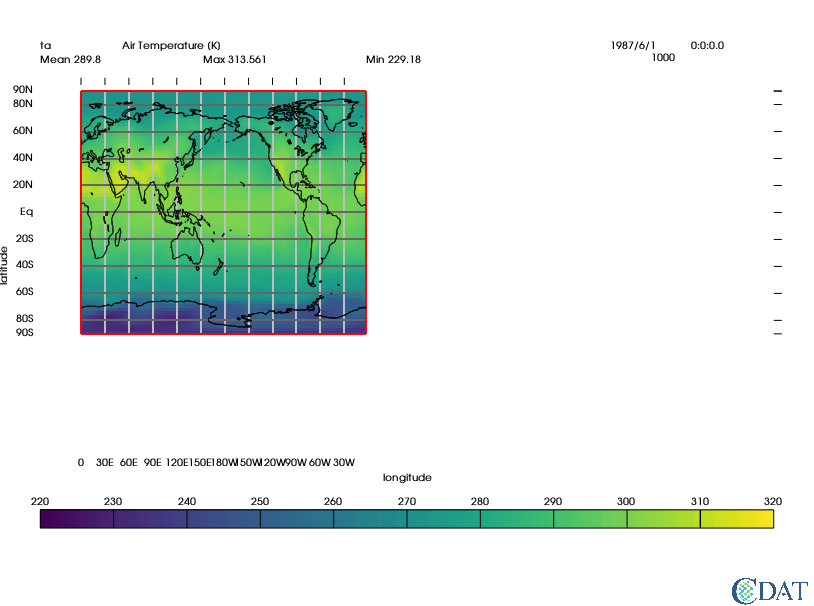##### Second set of major ticks (x/y)¶
In :
# x major tick marks for second set of tick marks (2) drawn outward in red
template.xtic2.priority=1  # turn on
template.xtic2.y1 = template.data.y2
template.xtic2.y2 = template.data.y2 + .01 # extend a bit further
template.xtic2.line="red"

# y major for second set of tick marks (2) drawn outward in red
template.ytic2.priority=1  # turn on
template.ytic2.x1 = template.data.x2
template.ytic2.x2 = template.data.x2 + .01 # extends a bit past it
template.ytic2.line = "red"
x.clear()
x.plot(data, template)

Out: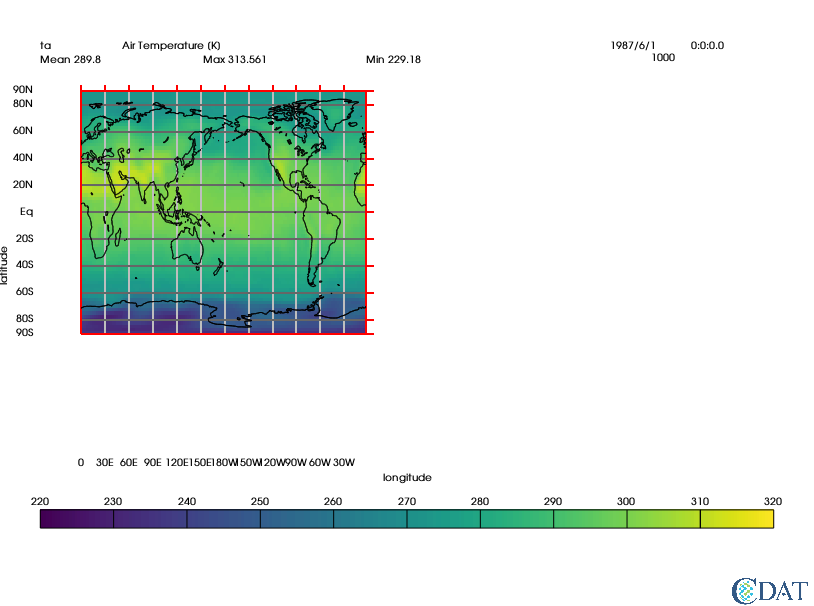#### Minor ticks (x/y)¶

Back to top

Let's do the same for the minor tics or mintics, except using dotted lines and not extending them as far.

In :
# x minor tick marks (set 1) drawn all the way across with grey lines
template.xmintic1.priority = 1
template.xmintic1.y1 = template.data.y1
template.xmintic1.y2 = template.data.y2
grey = vcs.createline(source=grey.name)
grey.type= ["dot",]
template.xmintic1.line = grey  # re-uses line object

# y minor (set 1) drawn all the way across using darker grey lines
template.ymintic1.priority = 1
template.ymintic1.x1 = template.data.x1
template.ymintic1.x2 = template.data.x2
grey2 = vcs.createline(source=grey2.name)
grey2.type= ["dot",]
template.ymintic1.line = grey2.name  # uses line name

# x minor tick marks (set 2) drawn outward in red (a bit less)
template.xmintic2.priority=1  # turn on
template.xmintic2.y1 = template.data.y2
template.xmintic2.y2 = template.data.y2 + .005 # extend a bit further
template.xmintic2.line="red"

# y minor (set 2) drawn outward in red
template.ymintic2.priority=1  # turn on
template.ymintic2.x1 = template.data.x2
template.ymintic2.x2 = template.data.x2 + .005 # extends a bit past it
template.ymintic2.line = "red"

x.clear()
x.plot(data, template)

Out: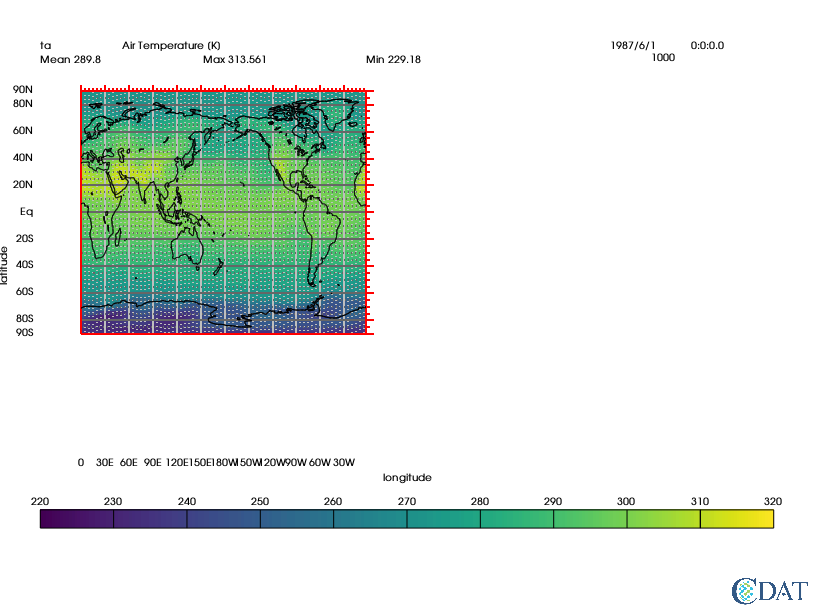In :
# too many tics; let's reduce their number
box = vcs.createboxfill()
box.xticlabels1 = {-180:"180W", -90:"90W", 0:"0", 90:"90E", 180:"180E", 270:"270E", 360:"0"}
box.xticlabels2 = box.xticlabels1 # same on both ends
box.yticlabels1 = {-90:"90S", -60:"60S", 0:"Eq", 60:"60N", 90:"90N"}
box.yticlabels2 = box.yticlabels1

# min ticks
box.xmtics1 = {-135:"135W", -45:"45W", 45:"45E", 135:"135E", 315:"315E"}
box.xmtics2 = box.xmtics1
box.ymtics1 = {-45:"45S", 45:"45N"}  # text does not matter as not drawn
box.ymtics2 = box.ymtics1

x.clear()
x.plot(data, box, template)

Out: